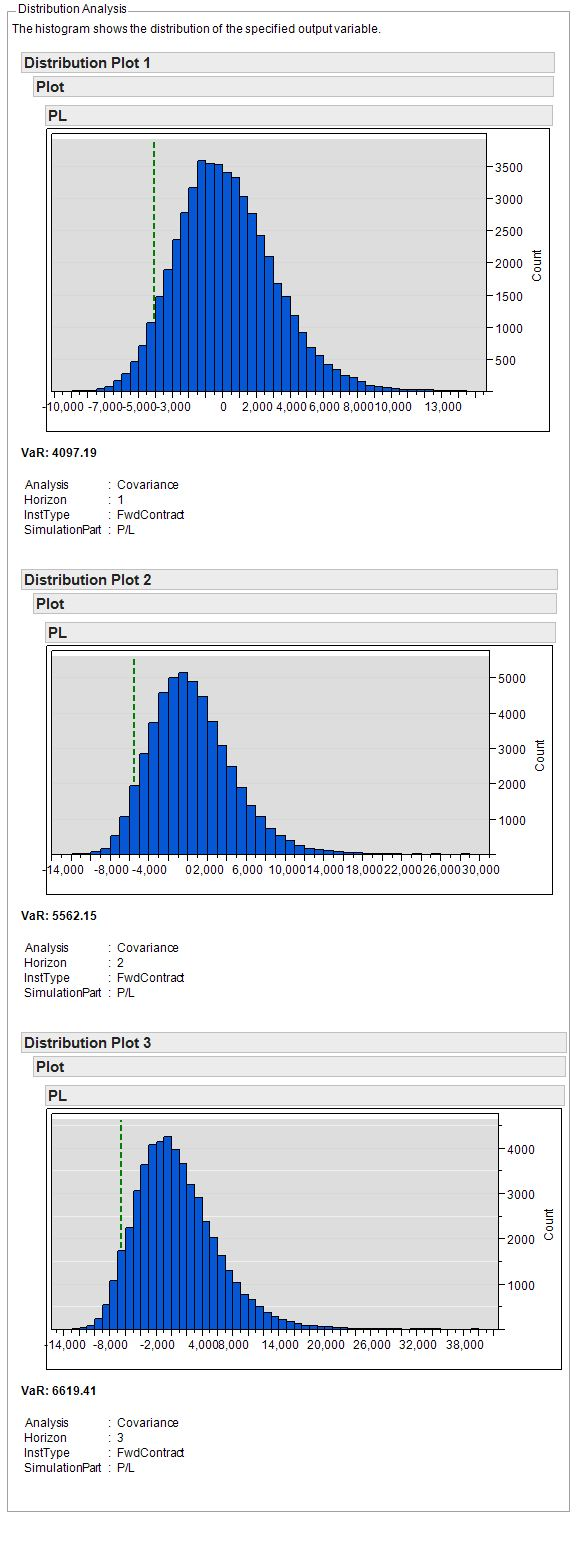# SAS Products

## SAS Risk Dimensions - Assessing Credit Risk

### Objective

In this exercise, you examine credit risk, which is the risk of losing money as a function of the creditworthiness of a counterparty. Specifically, you assess the credit risk of a simple forward agreement. A company has agreed to purchase 1000 shares of TechCompany stock at \$20 per share from CounterpartyA in July 2014. CounterpartyA is considered a midgrade company with a current credit rating of BBB.

To assess the risk, you simulate 50,000 market states and value the portfolio at each market state over a three-day horizon. Risk measures are computed from the distribution of values. For each market state you calculate the portfolio value, the profit/loss value, and the expected credit loss.

### Set Up Your Environment

First, set up the library for analysis and the name of the SAS Risk Dimensions environment. Use the directory C:\users\sasdemo\RD_Examples. You can assign the libref to any path as long as you have Write access to that directory.

libname RDExamp "C:\users\sasdemo\RD_Examples";
%let test_env = creditrisk;

In this code, you use the SAS Macro Language Facility to create the macro variable test_env. You assign the value creditrisk to the variable test_env. Using macro variables in this way gives you the flexibility to change the physical location of the target library and environment name in just two lines of code.

Next, create a new SAS Risk Dimensions environment assigned the name creditrisk in the library RDExamp.

proc risk;
env new=RDExamp.&test_env;
env save;
run;

### Register Your Portfolio Data

Next, define the assets in your portfolio and provide information about the counterparty. The following code creates a data set called instdata that stores information about the forward agreement in your portfolio. It specifies the characteristics of the instrument:

• counterparty name
• time to maturity
• interest rate
• contract type
• contract price
• holding

 data RDExamp.instdata; format maturity date9.; length insttype \$12. cpty_name \$16. contract_type \$16.; input insttype \$ instid \$ cpty_name \$ CounterpartyID \$ maturity date9. int_rate contract_type \$ contract_price holding; datalines; FwdContract instid1 CounterPartyA cptyid1 01JUL2014 0.02 TechCompany 20 1000 ;

The following code creates the data set cptydata that stores information about the counterparty, including the counterparty's current credit rating, which is BBB.

 data RDExamp.cptydata; length CounterPartyType \$16. cpartyname \$16. rating \$3.; input CounterPartyType \$ CounterpartyID \$ cpartyname \$ rating \$; datalines; MidGrade_Cpty cptyid1 CounterPartyA BBB ;

The following code registers the instrument data and counterparty data in the environment. It also defines the output variable creditloss, the value of which is computed in the pricing method.

proc risk;
env open=RDExamp.&test_env;
instdata Instruments file=RDExamp.instdata format=simple;
cptydata Counterparties file=RDExamp.cptydata;
declare
instvars = (
cpty_name char 16 var,
maturity num var,
int_rate num var,
contract_type char 16 var,
contract_price num var
)
cptyvars = (
rating char 3 var,
cpartyname char 16 var,
prob_default num computed,
loss_default num computed
)
outvars = (creditloss num computed comptype = exposure)
;
env save;
run;

### Register Market Data

Next, identify the risk factors that affect the value of your portfolio and the creditworthiness of the counterparty. Define current risk factor values and the variance-covariance values of the risk factors. Register the market data in the environment.

data RDExamp.mktdata;
TEC = 19.75;
run; data RDExamp.covdata;
length _name_ _type_ \$8;
_type_ = "COV";
input _name_ \$ TEC ;
datalines;
 TEC   .02
;

proc risk;
env open=RDExamp.&test_env;
declare riskfactors =(TEC num var label = "TechCompany Share Price");
marketdata Market file=RDExamp.mktdata type=current;
marketdata Cov file=RDExamp.covdata type=covariance;
env save;
run;

### Define Valuation Methods

Next, create both a scoring method and a pricing method. The scoring method is used to calculate probability of default (PD) and loss given default (LGD) based on the current credit rating of the counterparty. The outputs of the scoring method are then used by the pricing method to calculate the output variable creditloss.

Create methods using the COMPILE procedure, which is similar to and supports the functionality and syntax of the FCMP procedure. For more information, see the SAS Risk Dimensions: Users Guide.

proc compile env=RDExamp.&test_env outlib=RDExamp.&test_env;
method Score_Method kind = scoring;
if rating = 'BBB' then do;
prob_default = .10;
loss_default = .20;
end;
else do; prob_default = .15; loss_default = 1; end;
endmethod;

method Fwd_Contract kind=price;
time_yrs = (maturity - _date_)/365.25;
curr_fwd = TEC * exp(int_rate * time_yrs);
_value_ = (curr_fwd - contract_price)*exp(-1 * int_rate * time_yrs);
creditloss = max(0,_value_) * loss_default * prob_default ;
endmethod;
run;

Use the COUNTERPARTY statement of the RISK procedure to assign the scoring method to the counterparty type MidGrade_Cpty. Identify the counterparty variables needed for scoring by specifying the variables option. Use the INSTRUMENT statement to assign the pricing method to the instrument type FwdContract and to identify the instrument variables needed for pricing.

proc risk;
env open=RDExamp.&test_env;
counterparty MidGrade_Cpty variables = (rating, prob_default, loss_default)
method = Score_Method;
instrument FwdContract variables=(int_rate, maturity, contract_price,
counterpartyid, holding)
methods=(Price Fwd_Contract);
env save;
run;

### Specify the Analysis and Create the Project

Set up a project to simulate values for your portfolio based on the current market data and the covariance data. The following code

• creates a portfolio file from the instrument data set
• identifies the cross classifications for portfolio aggregation
• creates a covariance simulation analysis
• creates the project, specifying the portfolio and assigning the covariance simulation analysis

You need to specify the output variables in the simulation because only Profit/Loss is output by default. Also, to simulate values through time, specify horizons using the HORIZONS option. Specifying HORIZONS = (1 2 3) simulates values one, two, and three weekdays from the base case date. Note that you are not specifying a run date, so the base case date is today and horizon one is the following business day.

proc risk;
env open=RDExamp.&test_env;
sources DataSources (Instruments Counterparties);
crossclass CreditRiskCC (Insttype);
simulation Covariance method=covariance
ndraws=50000
outvars=(pl exposure creditloss)
horizon = (1 2 3)
data=Cov;
project Project
analysis=(Covariance)
portfolio=Portfolio
crossclass=CreditRiskCC
data=( Market );
env save;
run;

### Run the Project

Run the project, putting output files in the credit subdirectory of the environment.

proc risk;
env open=RDExamp.&test_env; runproject Project
out= Credit
options=(outall); env save;
run;

### View Results

Next, analyze your results. You can perform analysis on SAS data sets output from the project and you can view results graphically through the SAS Risk Dimensions GUI. Here is a brief introduction to the analysis tools. For further information, see the SAS Risk Dimensions: Users Guide.

The following figure shows the SimStat data set. It contains the simulation statistics for the portfolio such as Value at Risk, Expected Shortfall, and Mark to Market.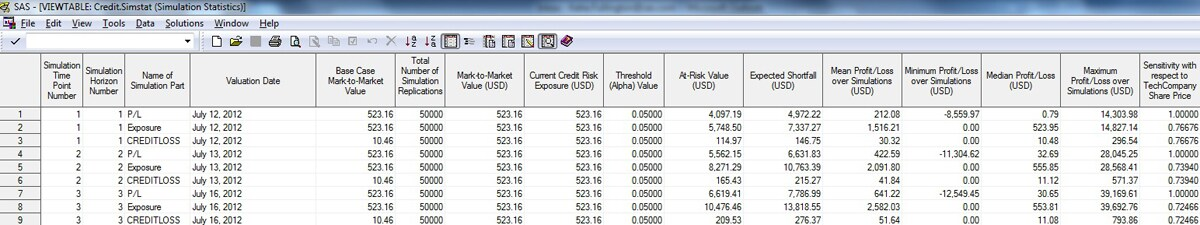The following figure shows the SimValue data set. This data set contains the simulated portfolio values.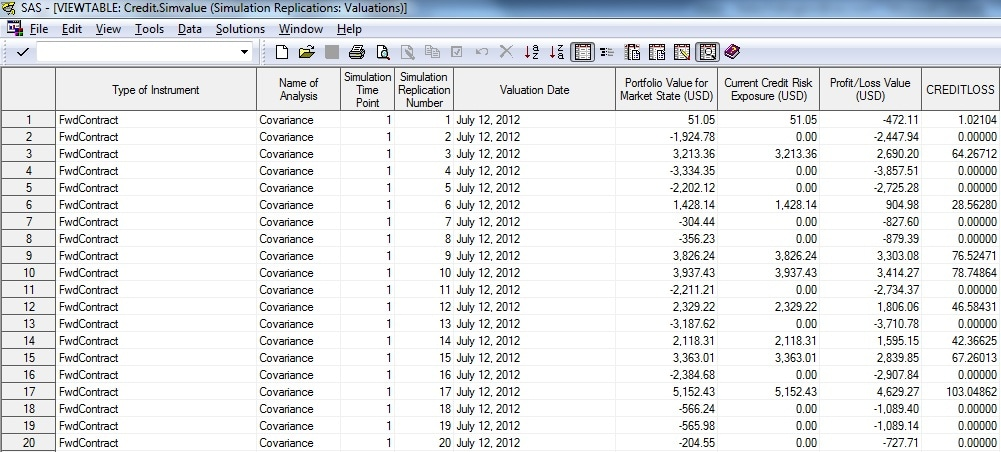You can explore the results of your project with the SAS Risk Dimensions GUI. From here you can view data sets and perform analysis with JMP. For more information, see the SAS Risk Dimensions: Users Guide.

The figures that follow demonstrate several of the built-in JMP scripts included in the SAS Risk Dimensions UI. You can use the JMP menu items to further customize these plots to your needs.

The plot below shows a histogram of simulated values of the CreditLoss output variable for the third horizon.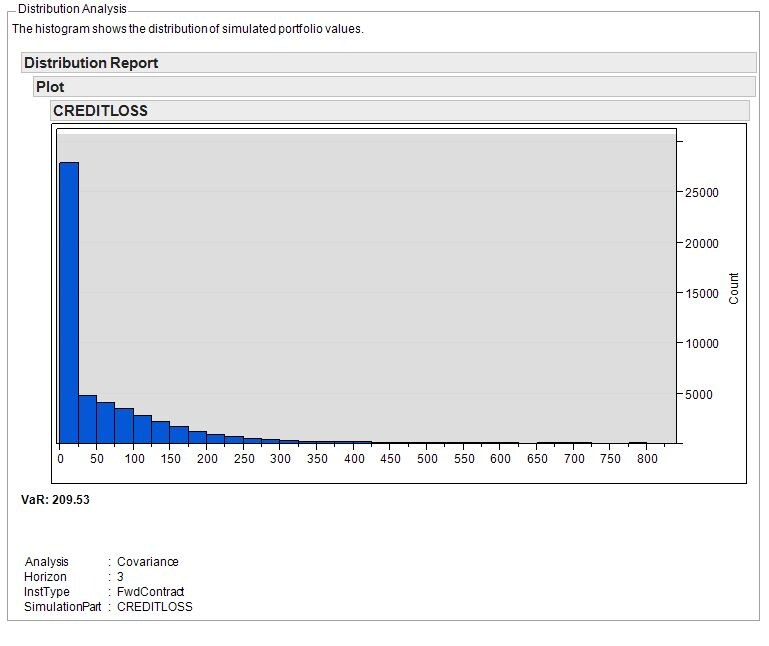The plot below compares the mean value of CreditLoss across the simulation horizons.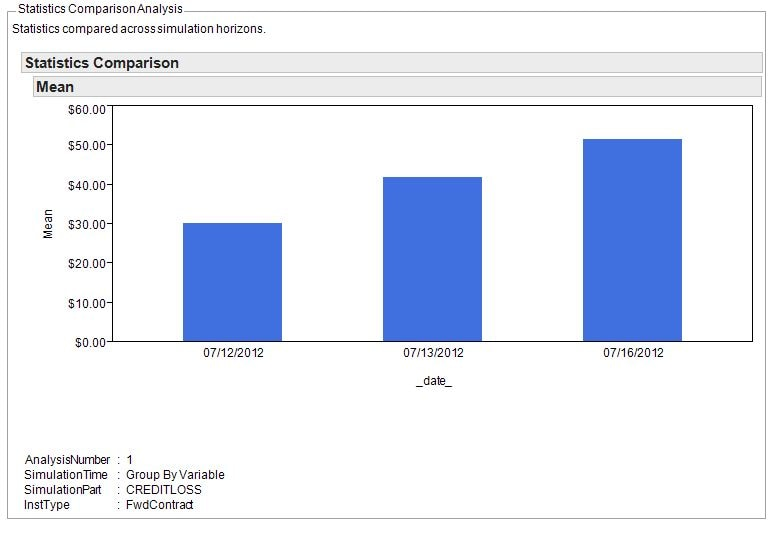The plot below compares the CreditLoss value at risk across simulation horizons.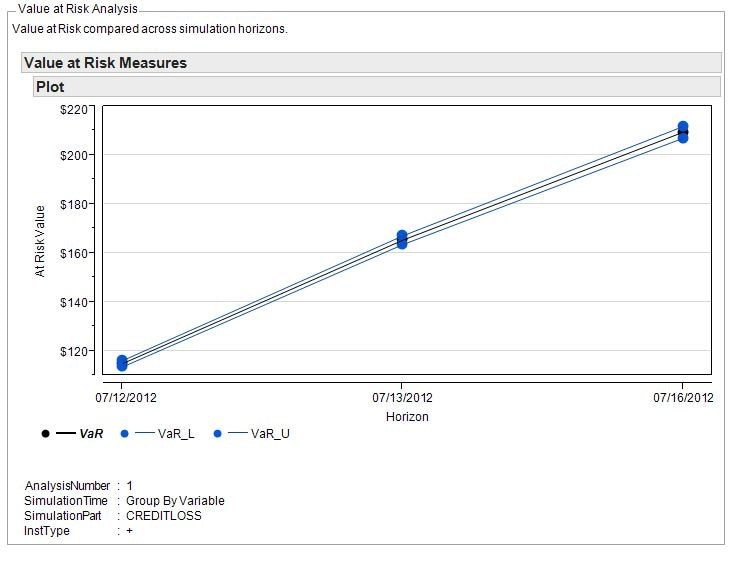The plot below compares the distribution of Profit/Loss for each simulation horizon.